This book is archived and will be removed July 6, 2022. Please use the updated version.

The Second Law of Thermodynamics

# 26 Entropy

### Learning Objectives

By the end of this section you will be able to:

• Describe the meaning of entropy
• Calculate the change of entropy for some simple processes

The second law of thermodynamics is best expressed in terms of a change in the thermodynamic variable known as entropy, which is represented by the symbol S. Entropy, like internal energy, is a state function. This means that when a system makes a transition from one state into another, the change in entropy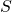is independent of path and depends only on the thermodynamic variables of the two states.

We first considerfor a system undergoing a reversible process at a constant temperature. In this case, the change in entropy of the system is given by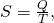where Q is the heat exchanged by the system kept at a temperature T (in kelvin). If the system absorbs heat—that is, with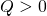—the entropy of the system increases. As an example, suppose a gas is kept at a constant temperature of 300 K while it absorbs 10 J of heat in a reversible process. Then from (Figure), the entropy change of the gas is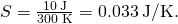Similarly, if the gas loses 5.0 J of heat; that is,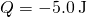, at temperature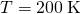, we have the entropy change of the system given by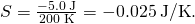Entropy Change of Melting Ice Heat is slowly added to a 50-g chunk of ice at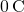until it completely melts into water at the same temperature. What is the entropy change of the ice?

Strategy Because the process is slow, we can approximate it as a reversible process. The temperature is a constant, and we can therefore use (Figure) in the calculation.

Solution The ice is melted by the addition of heat: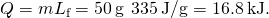In this reversible process, the temperature of the ice-water mixture is fixed at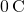or 273 K. Now from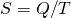, the entropy change of the ice is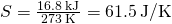when it melts to water at.

Significance During a phase change, the temperature is constant, allowing us to use (Figure) to solve this problem. The same equation could also be used if we changed from a liquid to a gas phase, since the temperature does not change during that process either.

The change in entropy of a system for an arbitrary, reversible transition for which the temperature is not necessarily constant is defined by modifying. Imagine a system making a transition from state A to B in small, discrete steps. The temperatures associated with these states are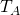and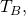respectively. During each step of the transition, the system exchanges heat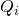reversibly at a temperature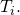This can be accomplished experimentally by placing the system in thermal contact with a large number of heat reservoirs of varying temperatures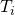, as illustrated in (Figure). The change in entropy for each step is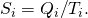The net change in entropy of the system for the transition is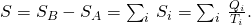We now take the limit as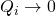, and the number of steps approaches infinity. Then, replacing the summation by an integral, we obtain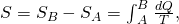where the integral is taken between the initial state A and the final state B. This equation is valid only if the transition from A to B is reversible.

The gas expands at constant pressure as its temperature is increased in small steps through the use of a series of heat reservoirs.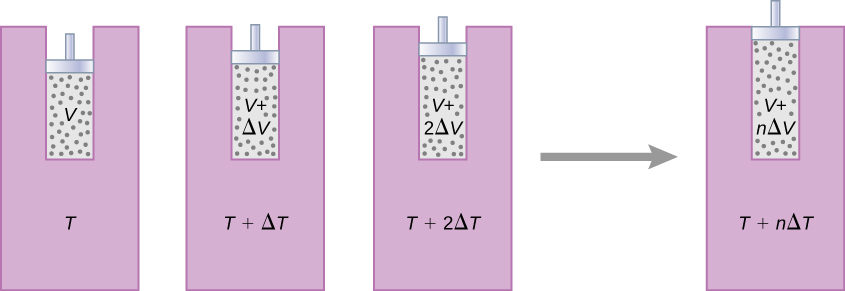As an example, let us determine the net entropy change of a reversible engine while it undergoes a single Carnot cycle. In the adiabatic steps 2 and 4 of the cycle shown in (Figure), no heat exchange takes place, so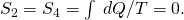In step 1, the engine absorbs heat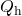at a temperature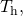so its entropy change is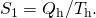Similarly, in step 3,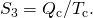The net entropy change of the engine in one cycle of operation is then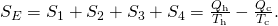However, we know that for a Carnot engine,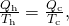so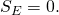There is no net change in the entropy of the Carnot engine over a complete cycle. Although this result was obtained for a particular case, its validity can be shown to be far more general: There is no net change in the entropy of a system undergoing any complete reversible cyclic process. Mathematically, we write this statement as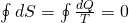where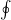represents the integral over a closed reversible path.

We can use (Figure) to show that the entropy change of a system undergoing a reversible process between two given states is path independent. An arbitrary, closed path for a reversible cycle that passes through the states A and B is shown in (Figure). From (Figure),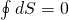for this closed path. We may split this integral into two segments, one along I, which leads from A to B, the other along II, which leads from B to A. Then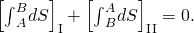Since the process is reversible,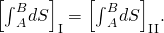The closed loop passing through states A and B represents a reversible cycle.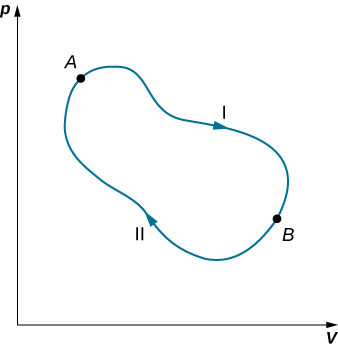Hence, the entropy change in going from A to B is the same for paths I and II. Since paths I and II are arbitrary, reversible paths, the entropy change in a transition between two equilibrium states is the same for all the reversible processes joining these states. Entropy, like internal energy, is therefore a state function.

What happens if the process is irreversible? When the process is irreversible, we expect the entropy of a closed system, or the system and its environment (the universe), to increase. Therefore we can rewrite this expression as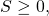where S is the total entropy of the closed system or the entire universe, and the equal sign is for a reversible process. The fact is the entropy statement of the second law of thermodynamics:

Second Law of Thermodynamics (Entropy statement)

The entropy of a closed system and the entire universe never decreases.

We can show that this statement is consistent with the Kelvin statement, the Clausius statement, and the Carnot principle.

Entropy Change of a System during an Isobaric Process Determine the entropy change of an object of mass m and specific heat c that is cooled rapidly (and irreversibly) at constant pressure from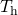to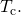Strategy The process is clearly stated as an irreversible process; therefore, we cannot simply calculate the entropy change from the actual process. However, because entropy of a system is a function of state, we can imagine a reversible process that starts from the same initial state and ends at the given final state. Then, the entropy change of the system is given by (Figure),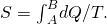Solution To replace this rapid cooling with a process that proceeds reversibly, we imagine that the hot object is put into thermal contact with successively cooler heat reservoirs whose temperatures range fromtoThroughout the substitute transition, the object loses infinitesimal amounts of heat dQ, so we have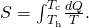From the definition of heat capacity, an infinitesimal exchange dQ for the object is related to its temperature change dT by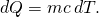Substituting this dQ into the expression for, we obtain the entropy change of the object as it is cooled at constant pressure from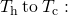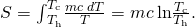Note that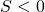here because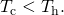In other words, the object has lost some entropy. But if we count whatever is used to remove the heat from the object, we would still end up with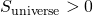because the process is irreversible.

Significance If the temperature changes during the heat flow, you must keep it inside the integral to solve for the change in entropy. If, however, the temperature is constant, you can simply calculate the entropy change as the heat flow divided by the temperature.

Stirling Engine The steps of a reversible Stirling engine are as follows. For this problem, we will use 0.0010 mol of a monatomic gas that starts at a temperature of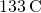and a volume of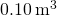, which will be called point A. Then it goes through the following steps:

1. Step AB: isothermal expansion atfromto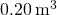2. Step BC: isochoric cooling to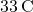3. Step CD: isothermal compression atfromto4. Step DA: isochoric heating back toand(a) Draw the pV diagram for the Stirling engine with proper labels.

(b) Fill in the following table.

Step W (J) Q (J)(J/K)
Step AB
Step BC
Step CD
Step DA
Complete cycle

(c) How does the efficiency of the Stirling engine compare to the Carnot engine working within the same two heat reservoirs?

Strategy Using the ideal gas law, calculate the pressure at each point so that they can be labeled on the pV diagram. Isothermal work is calculated using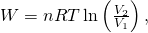and an isochoric process has no work done. The heat flow is calculated from the first law of thermodynamics,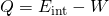where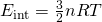for monatomic gasses. Isothermal steps have a change in entropy of Q/T, whereas isochoric steps have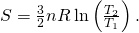The efficiency of a heat engine is calculated by using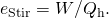Solution

1. The graph is shown below.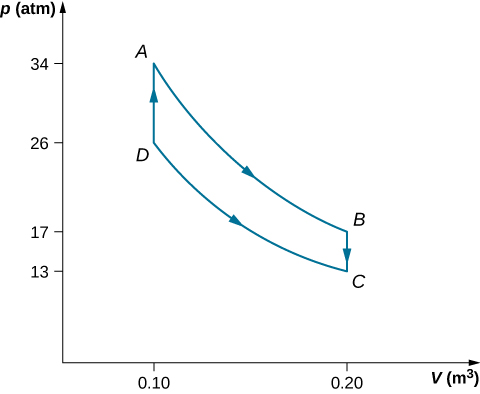2. The completed table is shown below.

Step W (J) Q (J)(J/K)
Step AB Isotherm 2.3 2.3 0.0057
Step BC Isochoric 0 –1.2 0.0035
Step CD Isotherm –1.8 –1.8 –0.0059
Step DA Isochoric 0 1.2 –0.0035
Complete cycle 0.5 0.5 ~ 0
3. The efficiency of the Stirling heat engine is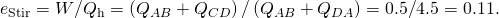If this were a Carnot engine operating between the same heat reservoirs, its efficiency would be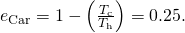Therefore, the Carnot engine would have a greater efficiency than the Stirling engine.

Significance In the early days of steam engines, accidents would occur due to the high pressure of the steam in the boiler. Robert Stirling developed an engine in 1816 that did not use steam and therefore was safer. The Stirling engine was commonly used in the nineteenth century, but developments in steam and internal combustion engines have made it difficult to broaden the use of the Stirling engine.

The Stirling engine uses compressed air as the working substance, which passes back and forth between two chambers with a porous plug, called the regenerator, which is made of material that does not conduct heat as well. In two of the steps, pistons in the two chambers move in phase.

### Summary

• The change in entropy for a reversible process at constant temperature is equal to the heat divided by the temperature. The entropy change of a system under a reversible process is given by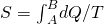.
• A system’s change in entropy between two states is independent of the reversible thermodynamic path taken by the system when it makes a transition between the states.

### Conceptual Questions

Does the entropy increase for a Carnot engine for each cycle?

Is it possible for a system to have an entropy change if it neither absorbs nor emits heat during a reversible transition? What happens if the process is irreversible?

Entropy will not change if it is a reversible transition but will change if the process is irreversible.

### Problems

Two hundred joules of heat are removed from a heat reservoir at a temperature of 200 K. What is the entropy change of the reservoir?

–1 J/K

In an isothermal reversible expansion at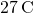, an ideal gas does 20 J of work. What is the entropy change of the gas?

An ideal gas at 300 K is compressed isothermally to one-fifth its original volume. Determine the entropy change per mole of the gas.

–13 J(K mole)

What is the entropy change of 10 g of steam at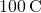when it condenses to water at the same temperature?

A metal rod is used to conduct heat between two reservoirs at temperatures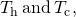respectively. When an amount of heat Q flows through the rod from the hot to the cold reservoir, what is the net entropy change of the rod, the hot reservoir, the cold reservoir, and the universe?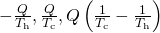For the Carnot cycle of (Figure), what is the entropy change of the hot reservoir, the cold reservoir, and the universe?

A 5.0-kg piece of lead at a temperature of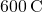is placed in a lake whose temperature is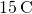. Determine the entropy change of (a) the lead piece, (b) the lake, and (c) the universe.

a. –540 J/K; b. 1600 J/K; c. 1100 J/K

One mole of an ideal gas doubles its volume in a reversible isothermal expansion. (a) What is the change in entropy of the gas? (b) If 1500 J of heat are added in this process, what is the temperature of the gas?

One mole of an ideal monatomic gas is confined to a rigid container. When heat is added reversibly to the gas, its temperature changes from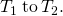(a) How much heat is added? (b) What is the change in entropy of the gas?

a.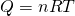; b.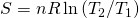(a) A 5.0-kg rock at a temperature of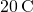is dropped into a shallow lake also atfrom a height of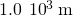. What is the resulting change in entropy of the universe? (b) If the temperature of the rock iswhen it is dropped, what is the change of entropy of the universe? Assume that air friction is negligible (not a good assumption) and that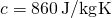is the specific heat of the rock.

### Glossary

entropy
state function of the system that changes when heat is transferred between the system and the environment
entropy statement of the second law of thermodynamics
entropy of a closed system or the entire universe never decreases